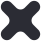Close

# Maths

Mathematics, at any level, requires complex problem-solving skills and the ability to consider a problem from another angle. The St Augustine’s maths department aim not only to teach curricular content, but to build on a student’s ability to think analytically and being able to apply that knowledge in a variety of different contexts.

At St Augustine’s, students develop as they progress through the years, building upon their current understanding to evaluate new concepts. They will cover the key concepts of number, algebra, geometry and data handling in all key stages. Each year the students will develop each concept further and this enables many students to progress to solving quadratic equations, sketching curves and using trigonometry to solve problems. Those students who decide to take Maths at A Level or Further Maths at GCSE will discover how calculus can be used to enhance their understanding of curves and how Mechanics can be used to model real world problems.

A strong grasp on maths will enable a student to think systematically and allow them access to a range of subject areas. As a maths teacher, I am constantly surprised by the variety and diversity of careers that our students go on to do, and hope that they can use the maths skills they have learnt at St Augustine’s as a tool for future success.

- Mr S Hunt, Head of Maths

### Key Stage 3

Year 7

In Year 7 the aim is to ensure that the students experience of transfer to secondary school is positive and challenging. Formal, whole class learning is introduced, especially of topics new to all. There is an intended overlap with the primary school syllabus on basic topics. Exercises are set on these basic topics to reassure, to build confidence and to challenge. Mathematical activities are planned which enable teachers to get to know students individually and which encourage students to work cooperatively. Emphasis, encouragement and support is given to the establishment of the standards of organisation (equipment, books, deadlines) and of presentation of written work that are expected in a secondary school. Year 7 students are divided into sets after 3 weeks of term 1. The initial setting of students is based on Key Stage 2 results and our own formative assessment.

Year 8 and 9

In Years 8 and 9 basic understanding and competence are established in fundamental mathematical topics – algebraic simplification, solving equations, using percentages and ratio, finding areas and volumes, drawing graphs, finding and using averages and calculating probability. Problem solving tasks and investigations are based on recognising patterns, solving puzzles and appreciating strategy in mathematical games. Tasks are chosen in which it is possible for the student to relate their solution to the underlying mathematical structure. The curriculum provides the foundation from which students tackle their GCSE course in Years 10 and 11.

In Year 8 the topics of number and data handling are followed by the autumn assessment. Measures, fractions, decimals, percentages and angles precede the spring assessment. Algebra, probability, number, transformations, ratio and proportion are followed by the summer assessment.

In Year 9 the number and angles topics are followed by the autumn assessment. Probability, number and algebra precede the spring assessment. Data handling, graphs, fractions, decimals, percentages, ratio, proportion and measures are followed by the summer assessment.

### GCSE

Mathematics is a core subject at GCSE and all students will take a full GCSE qualification or an Entry Level certificate. The Maths GCSE encourages students to develop confidence in, and a positive attitude towards, mathematics and to recognise the importance of mathematics in their own lives and to society. It prepares students to make informed decisions about the use of technology, the management of money, further learning opportunities and career choices.

What will I learn?

The students will develop their knowledge, skills and understanding of mathematical methods and concepts in the following areas:

• Number

• Algebra

• Geometry

• Measures

• Statistics and probability

They will use their knowledge and understanding to make connections between mathematical concepts and apply the functional elements of mathematics in everyday and real-life situations.

The content of the GCSE course is a natural progression from the Key Stage 3 syllabus. Topics covered in Year 9 will be revisited to ensure that progression through each unit of work is based on a solid foundation.

How will I be assessed?

The exam board is Edexcel (code: 1MA1) The GCSE is available at Higher tier (grades 9 - 4) and Foundation tier (grades 5 - 1).The assessment consists of three exam papers lasting ninety minutes which are taken at the end of Year 11. One exam paper is non-calculator. There is no controlled assessment.

The Entry Level Certificate is assessed using a series of tasks which are completed during normal lessons.